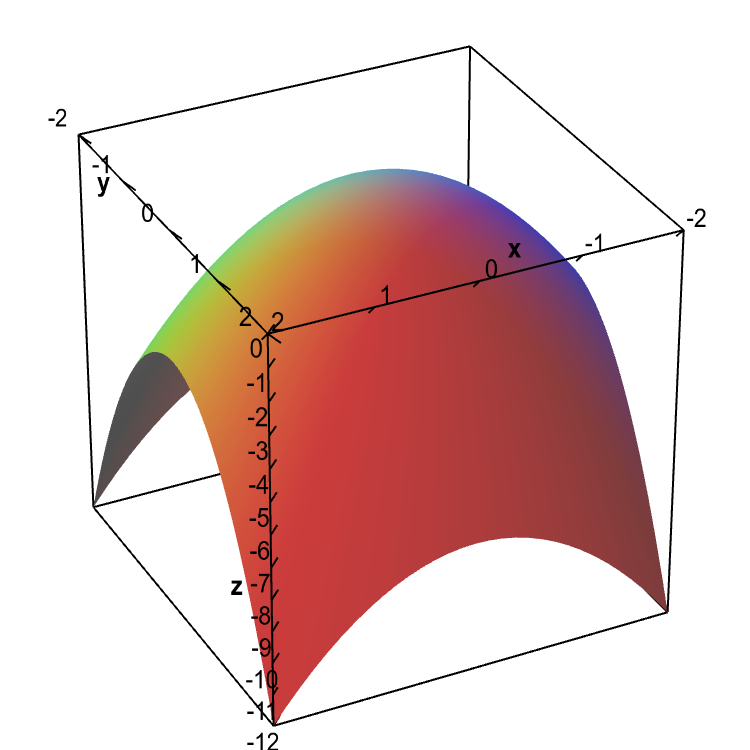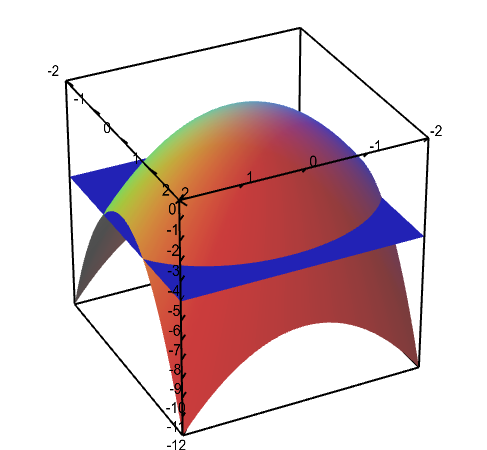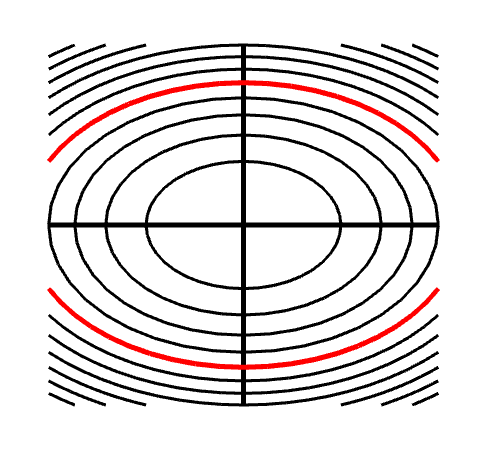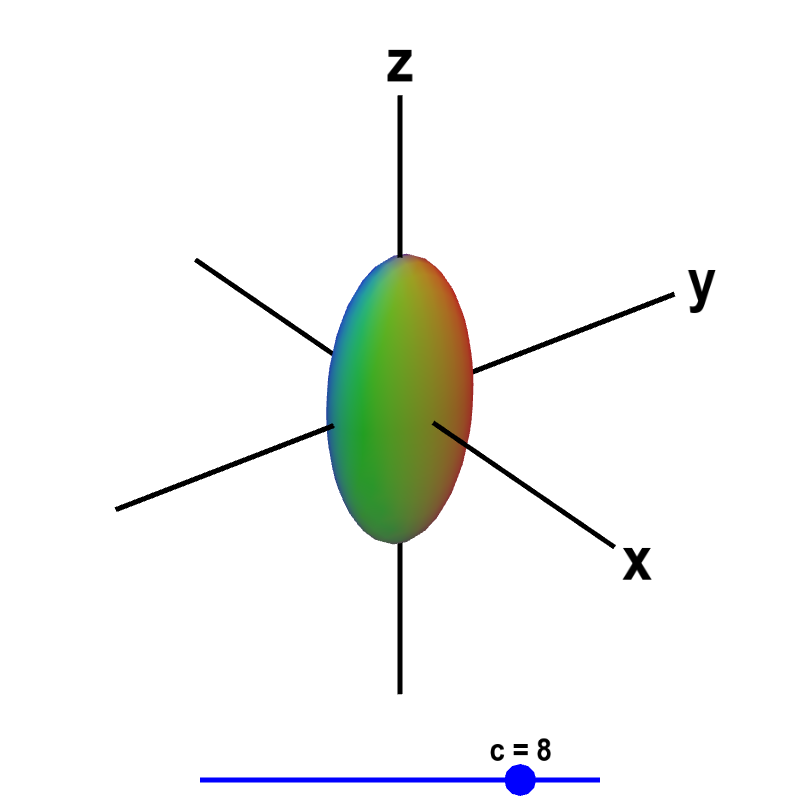# Math Insight

### Level sets

#### Graphing functions

One way to visualize functions is through their graphs. If $f(x,y)$ is a scalar-valued function of two variables, $f: \R^2 \to \R$ (confused?), then its graph is the surface formed by the set of all the points $(x,y,z)$ where $z=f(x,y)$, i.e., the set of points $(x,y,f(x,y))$. By graphing this surface, we can visualize the behavior of the function.

As an example, we graph the function $f(x,y)=-x^2-2y^2$ using the domain defined by $-2 \le x \le 2$ and $-2 \le y \le 2$. The graph of all points $(x,y,f(x,y))$ with $(x,y)$ in this domain is an elliptic paraboloid, as shown in the following figure.Graph of elliptic paraboloid. A graph of the function $f(x,y)=-x^2-2y^2$ over the domain $-2 \le x \le 2$ and $-2 \le y \le 2$.

Three-dimensional plots, such as the above figure, are more difficult to draw and visualize than two-dimensional plots. Moreover, the graph of a function $f(x,y,z)$ of three variables would be the set of points $(x,y,z,f(x,y,z))$ in four dimensions, and it would be difficult to imagine what such a graph would look like.

Another way of visualizing a function is through level sets, i.e., the set of points in the domain of a function where the function is constant. The nice part of of level sets is that they live in the same dimensions as the domain of the function. A level set of a function of two variables $f(x,y)$ is a curve in the two-dimensional $xy$-plane, called a level curve. A level set of a function of three variables $f(x,y,z)$ is a surface in three-dimensional space, called a level surface.

#### Level curves

One way to collapse the graph of a scalar-valued function of two variables into a two-dimensional plot is through level curves. A level curve of a function $f(x,y)$ is the curve of points $(x,y)$ where $f(x,y)$ is some constant value. A level curve is simply a cross section of the graph of $z=f(x,y)$ taken at a constant value, say $z=c$. A function has many level curves, as one obtains a different level curve for each value of $c$ in the range of $f(x,y)$. We can plot the level curves for a bunch of different constants $c$ together in a level curve plot, which is sometimes called a contour plot.

We return to the above example function $f(x,y) = -x^2-2y^2$. For some constant $c$, the level curve $f(x,y)=c$ is the graph of $c=-x^2-2y^2$. As long as $c<0$, this graph is an ellipse, as one can rewrite the equation for the level curve as \begin{align*} \frac{x^2}{-c} + \frac{y^2}{-c/2} =1. \end{align*} (If $c$ is negative, then both denominators are positive.) For example, if $c=-1$, the level curve is the graph of $x^2 + 2y^2=1$. In the level curve plot of $f(x,y)$ shown below, the smallest ellipse in the center is when $c=-1$. Working outward, the level curves are for $c=-2, -3, \ldots, -10$.

The below graph illustrates the relationship between the level curves and the graph of the function. The key point is that a level curve $f(x,y)=c$ can be thought of as a horizontal slice of the graph at height $z=c$. This slice is the intersection of the graph with the plane $z=c$.Level curves of an elliptic paraboloid shown with graph. The graph of the function $f(x,y)=-x^2-2y^2$ is shown is the first panel along with a level curve plot in the second panel. The level curve $f(x,y)=c$ is shown in red in the level curve plot, which is the same as the slice of the graph $z=f(x,y)$ by the plane $z=c$. You can change $c$ by dragging the plane slicing the graph up or down with the mouse. You can also change $c$ by dragging the red level curve.

Topographic maps are simply level curves of the elevation of the land (or water). For instance, on standard United States Geological Survey maps, each contour line represents 10 feet of elevation above sea level, as in this topographic map of Eagle Mountain, the highest mountain (well... hill) in Minnesota. The closer the contour lines are together, the steeper the slope of the land. Not surprisingly, the steepest slopes seem to be very near the summits of Eagle Mountain and Moose Mountain.

#### Level surfaces

For a scalar-valued functions of three variables, $f : \R^3 \to \R$, we would need four dimensions to draw its graph. The graph is the set of points $(x,y,z,f(x,y,z))$. You should familiarize yourself with vectors in higher dimensions to become comfortable with the idea of four dimensions. However, unless your mind is better at abstract visualization than most, you may have a hard time visualizing what this graph in four dimensions would look like.

Instead, we can look at the level sets where the function is constant. For a function of two variables, above, we saw that a level set was a curve in two dimensions that we called a level curve. For a function of three variables, a level set is a surface in three-dimensional space that we will call a level surface. For a constant value $c$ in the range of $f(x,y,z)$, the level surface of $f$ is the implicit surface given by the graph of $c=f(x,y,z)$.

The below version of the level curve applet will help you see that level curves and level surfaces are similar concepts, as this verion is put in the format that we will use for level surfaces. In this case, only one level curve $f(x,y)=c$ is displayed at a time.

A level curve of an elliptic paraboloid. A level curve of the function $f(x,y) = -x^2-2y^2=c$ is shown. You can drag the slider with the mouse to change $c$ and hence the level curve being displayed.

Now, we look at the function $f(x,y,z) = 10 e^{-9x^2-4y^2-z^2}$. Even though we can't plot the graph of $f(x,y,z)$ (without using four dimensions), we can still visualize the behavior of the function by plotting its level surfaces. We simply plot $f(x,y,z)=c$ for $c$ between 0 and 10. (Note that $f(x,y,z)$ always lies between $0$ and $10$. The argument of the exponential is always negative or zero, so the value of the exponential is always between zero and one.) The behavior of these level surfaces is illustrated in the following applet.Level surface of a function of three variables. A level surface $f(x,y,z) = 10 e^{-9x^2-4y^2-z^2}=c$ is shown. You can drag the blue point on the slider with the mouse to change $c$, and hence the level surface being displayed.
The significance of the level surfaces may become clearer if you think of $f(x,y,z)$ as giving the temperature at the point $(x,y,z)$. Then, the level surface $f(x,y,z)=2$ is the surface of points where the temperature is 2. In this example, the temperature at the origin is 10 and every other point has a lower temperature. Hence, the level surface $f(x,y,z)=c$ shrinks to a point around the origin as $c$ increases to 10.
As one moves away from the origin, the temperature decreases. The temperature decreases toward zero as you get far from the origin. The level surface $f(x,y,z)=0$ doesn't exist because $f(x,y,z)$ never reaches zero. But, if $c$ is a small positive number, the level surface $f(x,y,z)=c$ is a large ellipsoid. You can look at a couple more examples.